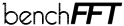Go back to the benchFFT results page.

# FFT Benchmark Methodology

Note that we only currently benchmark single-processor performance, even on multi-processor systems.

## Data formats

### Complex transforms

Most FFT routines in the benchmark accept complex data in interleaved format, i.e., as arrays of complex numbers. Some routines accept complex data in split format, i.e., as two separate arrays containing the real and imaginary part of the data. Some other routines accept either format.

Routines that accept a single format were benchmarked with that format. Routines that accept either format were benchmarked with interleaved data, which seems to be the most commonly used.

In interpreting the benchmark results, please notice that different formats are not strictly comparable.

### Real transforms

Each FFT routine seems to have its own way of storing the conjugate-symmetric output of real transforms, especially for multidimensional transforms. We benchmarked each routine using whatever format the routine chose to implement.

Again, routines that use different formats are not strictly comparable. Consequently, please interpret our plots only as a rough description of the relative merits of different codes. A faster code is of no use if it produces the output in a format that you do not want.

## Compilation

For each machine and compiler, we hand-pick "good" compiler options that seem to work well for optimized codes, which are then used for all codes, except for those that come with their own compilation scripts to pick their own optimization flags. Descriptions of the chosen compilers and flags are included in the results.

As far as possible, we use the unmodified, original codes, except where very minor patches were required for successful compilation. Rigorous correctness tests are performed to make sure that we are calling the FFT properly (and that it is bug-free).

## Timing

Our FFT timing measurement is intended to reflect the common case where many FFTs of the same size, indeed of the same array, are required. Thus, we break the measurement into two parts:

### Initialization

First, we call any separate initialization/setup routines provided by the code. This step may include calling the code's FFT once, if it performs initialization on the first call. This setup time is measured separately from the FFT performance below, but only as a rough indicator; no attempt is made to perform repeated measurements or to make our initialization preparations as efficient as possible.

### Performance

Second, we measure the FFT performance by performing repeated FFTs of the same zero-initialized array.

The input array is initialized to zero to prevent divergences from repeated FFTs of the same array. No FFT code that we know of, or any floating-point hardware in the benchmark, has a speed that depends on the input data (except for floating-point exceptions).

The timing procedure consists of two loops. First, we compute enough repeated FFTs so that the total time is sufficient for accurate timing, and divide by the number of iterations to obtain the average time. Second, we repeat this averaging process eight times, and report the minimum average time (to avoid fluctuations due to system interrupts, cache priming, etcetera).

The timer routine is `gettimeofday`, and the minimum time for accurate measurement is computed using a calibration routine from lmbench, a free Unix benchmark by Larry McVoy and Carl Staelin, who graciously permitted us to use their routine under the LGPL license.

## Reporting

We report the collected data in two formats: as graphs and as raw data files. You can view graphs for a selection of the results at this web page, and you can download the full raw data files from our ftp site.

### Plots

To report FFT performance, we plot the "mflops" of each FFT, which is a scaled version of the speed, defined by:

mflops = 5 N log2(N) / (time for one FFT in microseconds) for complex transforms, and
mflops = 2.5 N log2(N) / (time for one FFT in microseconds) for real transforms,
where N is number of data points (the product of the FFT dimensions). This is not an actual flop count; it is simply a convenient scaling, based on the fact that the radix-2 Cooley-Tukey algorithm asymptotically requires 5 N log2(N) floating-point operations. It allows us to compare the performance for many different sizes on the same graph, get a sense of the cache effects, and provide a rough measure of "efficiency" relative to the clock speed.

In order to keep the graphs readable, however, we only plot the "fastest" results. That is, when a particular code has several variants (different radices, calling options, etcetera), we only plot the variant that is "on average" the fastest (with a few exceptions, for codes of particular interest or for variants with radically different performance characteristics). We also only plot either the forward or the backward transform speed, whichever is faster "on average".

To quantify the "average" speed of a FFT routine, and also to reorder the plot legend for improved readability, we define a plot rank for each FFT as follows. First, for each transform size in a plot, we compute a rank = (mflops for FFT) / (mflops for fastest FFT for that size). Then, the plot rank of a given FFT is defined as the median of its ranks for all sizes in the plot. The plot rank is only a convenient heuristic to produce useful plots, and it should not be interpreted as an absolute measure of performance.

### Raw data files

In the raw benchmark data output, the speed for all routines, for both forward and backward transforms, is collected in the file `host.speed` in the space-delimited format:

`name-of-code transform-type transform-size mflops time setup-time`

where the times are in seconds.

transform-type is a four-character string consisting of precision (double/single = `d`/`s`), type (complex/real = `c`/`r`), in-place/out-of-place (= `i`/`o`), and forward/backward (= `f`/`b`). For example, transform-type = `dcif` denotes a double-precision in-place forward transform of complex data.

Go back to the benchFFT results page.